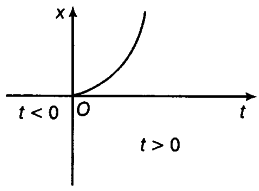# Figure shows x-t plot of one-dimensional motion of a particle

Figure shows x-t plot of one-dimensional motion of a particle. Is it correct to say from the graph that the particle moves in a straight line for t < 0 and on a parabolic path for t > 0? If not, suggest a suitable physical context in this graph.No, because the x-t graph does not represent the trajectory of the path followed by a particle. From the graph, it is noted that at t = 0, x = 0.
Context The above graph can represent the motion of a body falling freely from a tower under gravity.